Courses

# NCERT Summary: Summary of Physics- 2 UPSC Notes | EduRev

## UPSC : NCERT Summary: Summary of Physics- 2 UPSC Notes | EduRev

The document NCERT Summary: Summary of Physics- 2 UPSC Notes | EduRev is a part of the UPSC Course Famous Books for UPSC Exam (Summary & Tests).
All you need of UPSC at this link: UPSC

Light

• To understand light you have to know that what we call light is what is visible to us. Visible light is the light that humans can see. Other animals can see different types of light. Dogs can see only shades of gray and some insects can see light from the ultraviolet part of the spectrum.
• As far as we know, all types of light move at one speed when in a vacuum. The speed of light in a vacuum is 299,792,458 meters per second.
• Any medium through which light can travel is an optical medium. If this medium is such that light travels with equal speed in all directions, then the medium is called a homogeneous medium. The homogeneous media through which light can pass easily, are called transparent media. The media through which light cannot pass, are called opaque media. Again the media through which light can pass partly, are called translucent media.
• Light travels along a Straight Line: Light is reflected from all surfaces. Regular reflection takes place when light is incident on smooth, polished, and regular surfaces.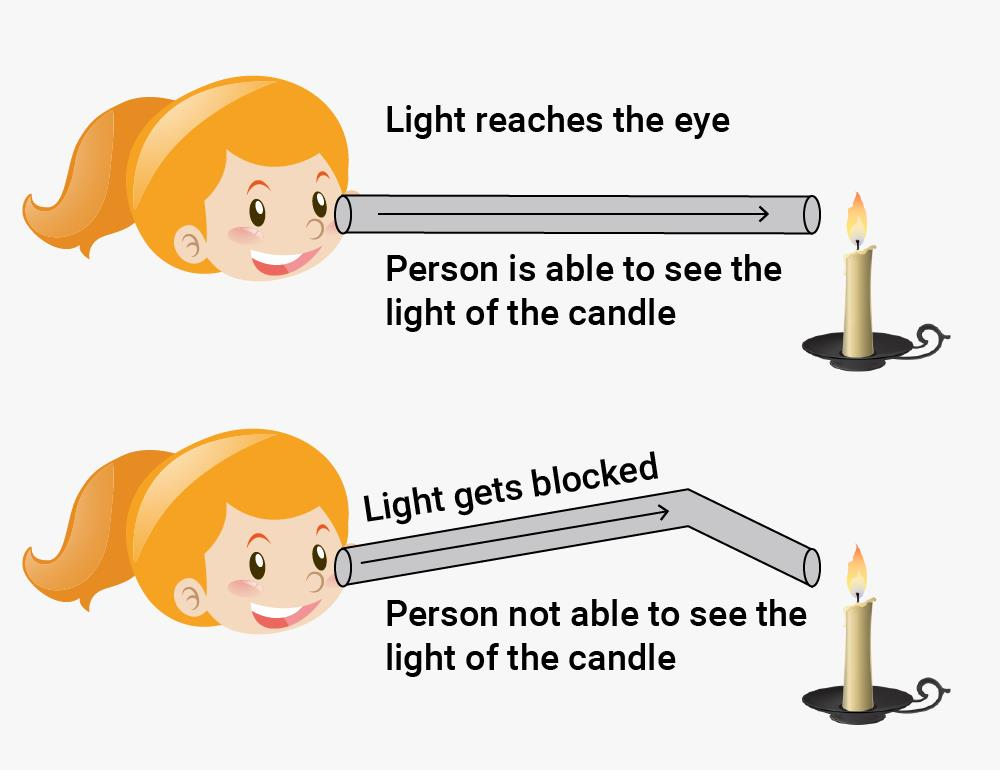• After striking the surface, the ray of light is reflected in another direction. The light ray, which strikes any surface, is called the incident ray. The ray that comes back from the surface after reflection is known as the reflected ray.
• The angle between the normal and incident ray is called the angle of incidence. The angle between the normal and the reflected ray is known as the angle of reflection.

Laws of Reflection

• Two laws of reflection are:
(i) The angle of incidence is equal to the angle of reflection.
(ii) Incident ray, reflected ray and the normal drawn at the point of incidence to the reflecting surface, lie in the same plane.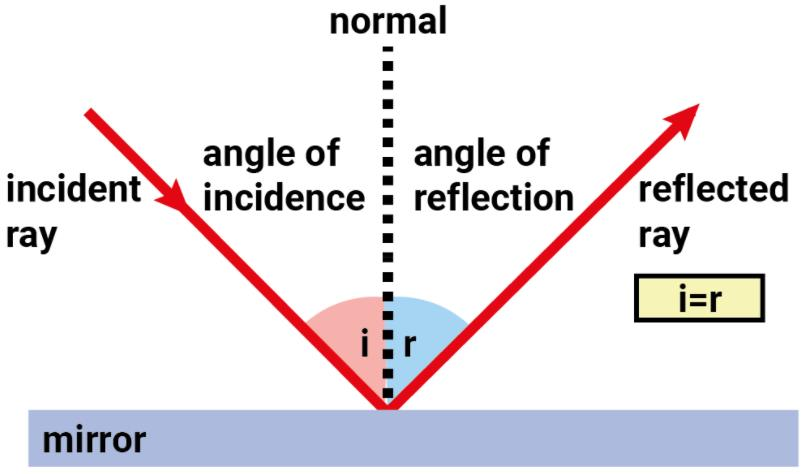• When all the parallel rays reflected from a plane surface are not parallel, the reflection is known as diffused or irregular reflection. On the other hand reflection from a smooth surface like that of a mirror is called regular reflection.
Types of Images
• When rays of light coming from a point of source, after reflection or refraction, actually meet at another point or appear to diverge from another point, the second point is called the image of the first point.
Images may be of two types:
(i) Real
(ii) Virtual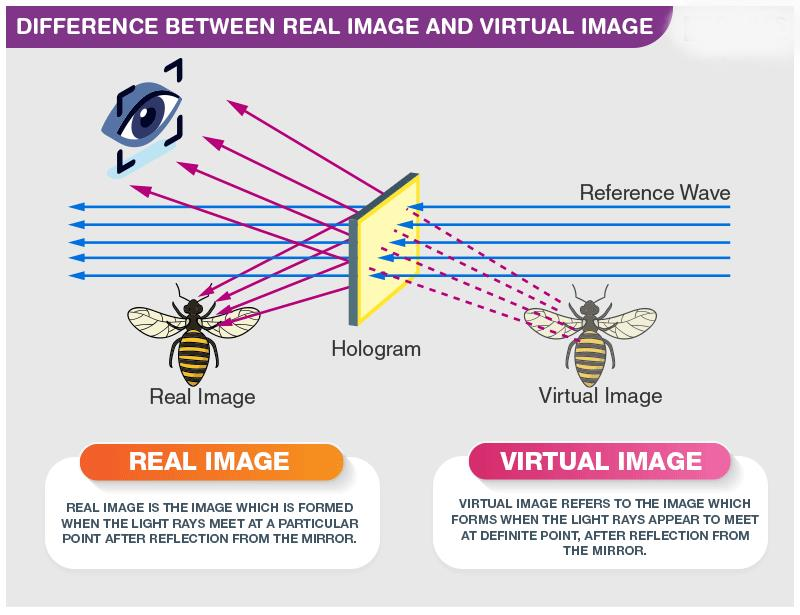• An image that can be obtained on a screen is called a real image. An image that cannot be obtained on a screen is called a virtual image.
• The image formed by a plane mirror is erect. It is virtual and is of the same size as the object. The image is at the same distance behind the mirror as the object is in front of it.
Types of Mirror
• The reflecting surface of a spherical mirror may be curved inwards or outwards. A spherical mirror, whose reflecting surface is curved inwards, that is, faces towards the center of the sphere, is called a concave mirror.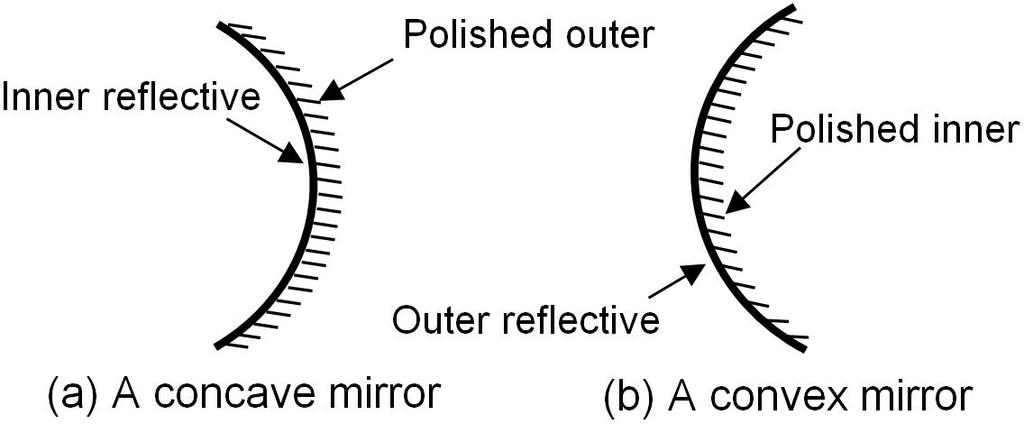• A spherical mirror whose reflecting surface is curved outwards is called a convex mirror.
• The center of the reflecting surface of a spherical mirror is a point called the pole. It lies on the surface of the mirror. The pole is usually represented by the letter P.
• The reflecting surface of a spherical mirror forms a part of a sphere. This sphere has a center. This point is called the center of curvature of the spherical mirror. It is represented by the letter C.

Please note that the center of curvature is not a part of the mirror. It lies outside its reflecting surface. The center of curvature of a concave mirror lies in front of it. However, it lies behind the mirror in case of a convex mirror.

• The radius of the sphere of which the reflecting surface of a spherical mirror forms a part is called the radius of curvature of the mirror. It is represented by the letter R. You may note that the distance PC is equal to the radius of curvature.
• Imagine a straight line passing through the pole and the center of curvature of a spherical mirror. This line is called the principal axis.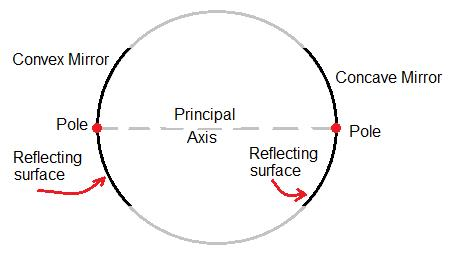Spherical Mirror
• Concave mirrors are commonly used in torches, search-lights, and vehicle headlights to get powerful parallel beams of light. They are often used as shaving mirrors to see a larger image of the face. The dentists use concave mirrors to see large images of the teeth of patients. Large concave mirrors are used to concentrate sunlight to produce heat in solar furnaces.
• Convex mirrors are commonly used as rear-view (wing) mirrors in vehicles. These mirrors are fitted on the sides of the vehicle, enabling the driver to see traffic behind him/her to facilitate safe driving. Convex mirrors are preferred because they always give an erect, though diminished, image. Also, they have a wider field of view as they are curved outwards. Thus, convex mirrors enable the driver to view much larger area than would be possible with a plane mirror.
Types of Lenses
• Lenses are widely used in spectacles, telescopes, and microscopes. Those lenses which feel thicker in the middle than at the edges are convex lenses. Those which feel thinner in the middle than at the edges are concave lenses. Notice that the lenses are transparent and light can pass through them.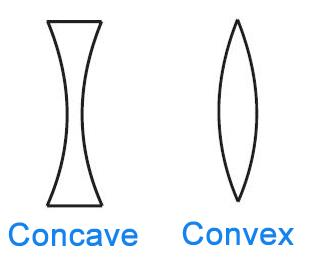Concave and Convex Lens
• A convex lens converges (bends inward) the light generally falling on it. Therefore, it is called a converging lens. On the other hand, a concave lens diverges (bends outward) the light and is called a diverging lens.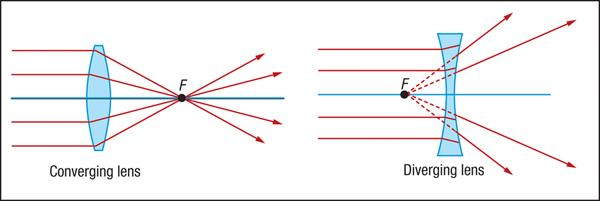• A convex lens can form a real and inverted image. When the object is placed very close to the lens, the image formed is virtual, erect and magnified. When used to see objects magnified, the convex lens is called a magnifying glass.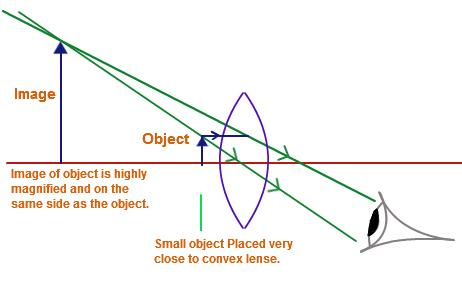Convex lens as a magnifying glass
• A concave lens always forms erect, virtual and smaller image than the object.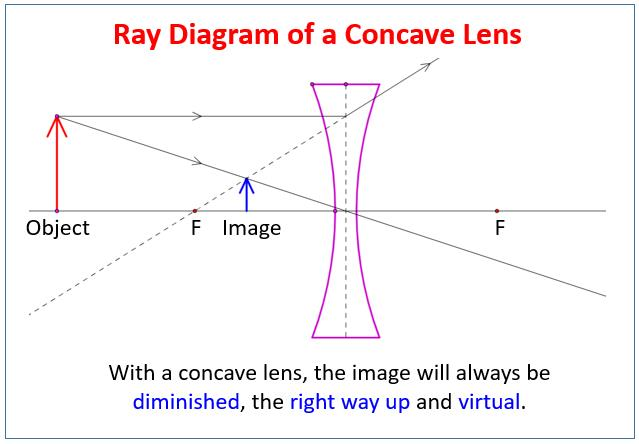• The two surfaces of the lens are parts of two spheres. The straight line joining obtained by joining two centers of the spheres is called the Principal axis. Generally, we use lenses whose surfaces have equal curvature. In such lenses, if we take a point on the principal axis inside the lens equidistant from the two surfaces, the point is called the optical center of the lens.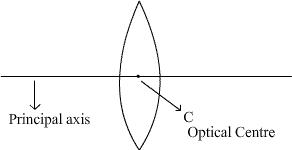Optical center and principal axis of a convex lens
• If a beam of parallel rays, traveling parallel to the principal axis of a convex lens, is refracted by the lens, the rays become converging and intersect each other at a particular point of the axis. The point is called the focus of the convex lens. The focal length of a lens is the distance between the optical center and the focus of the lens.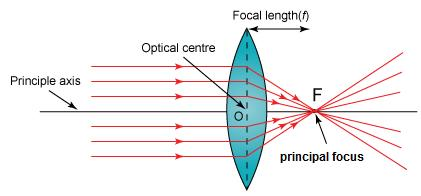Classification of solids on the basis of electricity conduction
• The power of a lens is a measure of the degree of convergence (in the case of a convex lens) or divergence (in the case of a concave lens). It is defined as the reciprocal of its focal length expressed in meters.
• The S.I. Unit of power of a lens is dioptre, the symbol being D. Thus, 1 dioptre is the power of a lens whose focal length is 1 meter. 1D = 1m–1

You may note that the power of a convex lens is positive and that of a concave lens is negative.

Refraction of Light
• The phenomenon due to which a ray of light deviates from its path, at the surface of separation of two media, when the ray of light is traveling from one optical medium to another optical medium is called refraction of light. When a ray of light travels from an optically rare medium to an optically denser medium.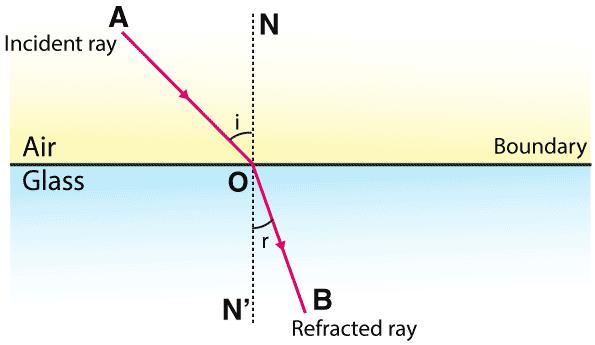Refraction of Light

1. Phenomenon of Refraction

• When a ray of light travels from an optically denser medium to an optically rare medium, it bends away from the normal at the surface of separation of two media.
• When a ray of light strikes the surface of separation of two media normally, it does not deviate from its original path. Some indexes of refraction are diamond (2.419), glass (1.523), and water (1.33).
• Total internal reflection is the phenomenon that involves the reflection of all the incident light off the boundary.
Total internal reflection only takes place when both of the following two conditions are met:
(i) The light is in the more dense medium and approaching the less dense medium.
(ii) The angle of incidence is greater than the so-called critical angle.
• Total internal reflection will not take place unless the incident light is traveling within the more optically dense medium towards the less optically dense medium.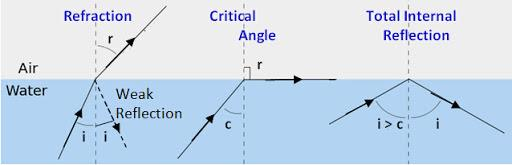The Phenomenon of Total Internal Reflection

2. Dispersion of Light

• It is the phenomenon splitting of a beam of white light into its constituent colors on passing through a prism. The order of colors from the lower end are violet, indigo, blue, green, yellow, orange, and red. At one end of the band, there is red and at the other violet. The sequence of colors can be best remembered by the word VIBGYOR’ which is formed by taking the initial letter of each color.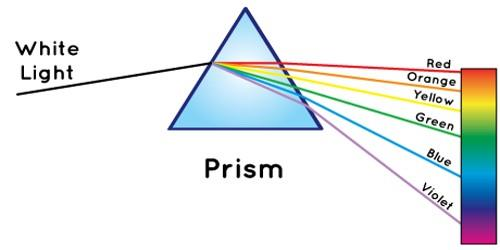Dispersion of Light
• A laser is just a really powerful beam of light. The laser isn’t a word but an acronym. It stands for Light Amplification By Stimulated Emission Of Radiation

Magnetism and Electricity
1. Magnetism
• The word magnet is derived from the name of an island in Greece called Magnesia where magnetic ore deposits were found, as early as 600 BC. Magnetite, an iron ore, is a natural magnet. It is called a lodestone.
• When a bar magnet is freely suspended, it points in the north-south direction. The tip which points to the geographic north is called the north pole and the tip which points to the geographic south is called the south pole of the magnet. There is a repulsive force when the north poles (or south poles) of two magnets are brought close together. Conversely, there is an attractive force between the north pole of one magnet and the south pole of the other.
• The properties of a magnet are:
(i) It attracts a small piece of iron towards it.
(ii) It always comes to rest in a north-south direction when suspended freely.
(iii) As poles repel, unlike poles attracts each other
(iv) Magnetic poles always exist in pairs.
(v) The strength of a magnet is maximum at poles located near the poles.
• The phenomenon due to which an unmagnetized magnetic substance behaves like a magnet, due to the presence of some other magnet, is called magnetic induction. Magnetic induction takes place first then magnetic attraction.

Magnetic Induction

• Magnetic induction depends upon the nature of the magnetic substance. Magnetic induction is inversely proportional to the distance between the inducing magnet and the magnetic substance. The more powerful the inducing magnet, the more strong will be the magnetism in magnetic substance.

Magnetic Field

• The space around the magnet where its influence can be detected is called the magnetic field.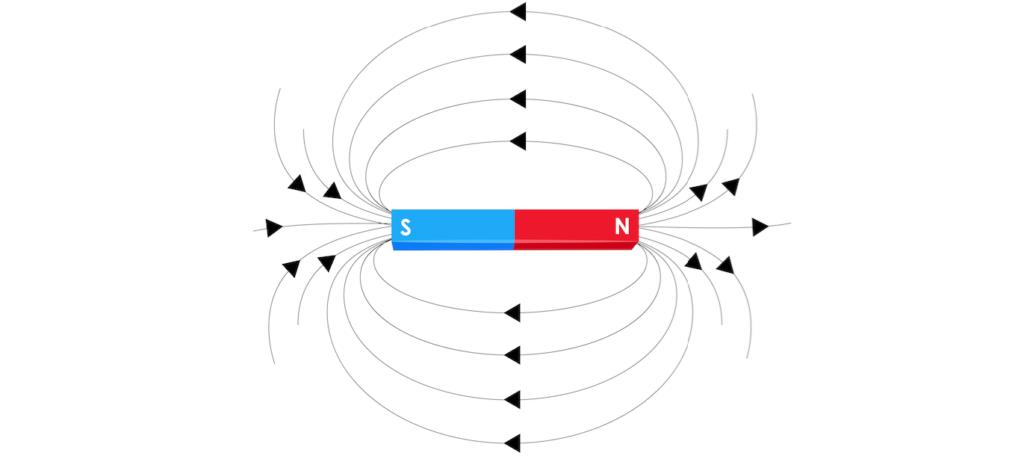Magnetic Field Around the Bar Magnet
• A curve in a magnetic field, along with a free north magnetic pole will move, is called magnetic line of force. The direction of magnetic lines of force is the direction in which the free north pole will move in a magnetic field.
(i) They travel from north to south pole outside the magnet and from south to north pole inside the magnet.
(ii) They mutually repel each other.
(iii) They never intersect with each other.
• The earth behaves as a magnet with the magnetic field pointing approximately from the geographic south to the north. At a particular place on earth, the magnetic north is not usually in the direction of the geographic north. The angle between the two directions called declination.
2. Electricity
• The phenomenon due to which a suitable combination of bodies on rubbing, get electrified is called electricity. If a charge on a body is not allowed to flow, it is called static electricity.
• Matters are made of atoms. An atom is basically composed of three different components — Electrons, Protons, and Neutrons. An electron can be removed easily from an atom. When two objects are rubbed together, some electrons from one object move to another object.
Example: When a plastic bar is rubbed with fur, electrons will move from the fur to the plastic stick. Therefore, plastic bar will be negatively charged and the fur will be positively charged.
• When two objects are rubbed together, some electrons from one object move to another object.
Example: When a plastic bar is rubbed with fur, electrons will move from the fur to the plastic stick. Therefore, plastic bar will be negatively charged and the fur will be positively charged.
• When you bring a negatively charged object close to another object, electrons in the second object will be repelled from the first object. Therefore, that end will have a negative charge. This process is called charging by induction.
• When a negatively charged object touches a neutral body, electrons will spread on both objects and make both objects negatively charged. This process is called charging by conduction. The other case, positively charged object touching the neutral body, is just the same in principle.

Types of Substance

• Substances can be classified into three typesInsulators, Conductors, and Semiconductors.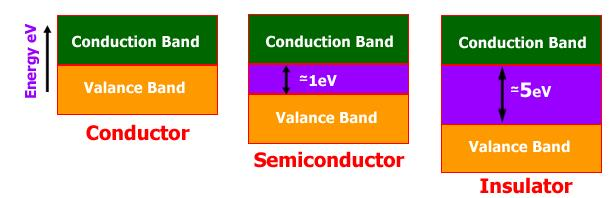Classification of Solids on the basis of Electricity Conduction
• Conductors are materials in which electrical charges and heat energy can be transmitted very easily. Almost all metals such as gold, silver, copper, iron, and lead are good conductors.
(i) Insulators are materials that allow very little electrical charges and heat energy to flow. Plastics, glass, dry air, and wood are examples of insulators.
(ii) Semiconductors are materials that allow the electrical charges to flow better than insulators, but less than conductors.
Example: Silicon and Germanium.

Types of Electric Charges

• There are two different types of electric charges namely the positive and negative charges. Like charges repel and unlike charges attract each other.
• Electric current always flows from the point of high potential. The potential difference between two conductors is equal to the work done in conducting a unit positive charges from one conductor to the other conductor through a metallic wire.
• The flow of charge is called the current and it is the rate at which electric charges pass through a conductor. The charged particle can be either positive or negative. In order for a charge to flow, it needs a push (a force) and it is supplied by voltage or potential difference. The charge flows from high potential energy to low potential energy.
• A closed-loop of current is called an electric circuit. The current [I] measures the amount of charge that passes a given point every second. The unit for current is Ampere [A]. 1 A means that 1 C of charge passes every second.
• When current flows through a conductor it offers some obstruction to the flow of current The obstruction offered to flow of current by the conducting wire is called its resistance in the passage of electricity.
• The unit of resistance is Ohm. The resistance varies in different materials.
Example: gold, silver, and copper have low resistance, which means that current can flow easily through these materials. Glass, plastics, and wood have very high resistance, which means that current can not pass through these materials easily.

Electromagnetism

• The branch of physics which deals with the relationship between electricity and magnetism is called electromagnetism.
• Whenever current is passed through a straight conductor it behaves like a magnet. The magnitude of magnetic effect increases with the increase in the strength of current.
• Faraday’s law of induction is one of the important concepts of electricity. It looks at the way changing magnetic fields can cause current to flow in wires. Basically, it is a formula/concept that describes how potential difference (voltage difference) is created and how much is created. It’s a huge concept to understand that the changing of a magnetic field can create voltage.
• He discovered that the changes in the magnetic field and the size of the field were related to the amount of current created. Scientists also use the term magnetic flux. Magnetic flux is a value that is the strength of the magnetic field multiplied by the surface area of the device.

Coulomb’s Law

• Coulomb’s Law is one of the basic ideas of electricity in physics. The law looks at the forces created between two charged objects. As distance increases, the forces and electric fields decrease. This simple idea was converted into a relatively simple formula. The force between the objects can be positive or negative depending on whether the objects are attracted to each other or repelled.
• Coulomb’s Law: When you have two charged particles, an electric force is created. If you have larger charges, the forces will be larger. If you use those two ideas, and add the fact that charges can attract and repel each other you will understand Coulomb’s Law.
• It’s a formula that measures the electrical forces between two objects.

= kq1q/ r2

» Where ”F” is the resulting force between the two charges.
» The distance between the two charges is ”r”. The “r” actually stands for “radius of separation” but you just need to know it is a distance.
» The ”q2" and ”q2" values for the amount of charge in each of the particles. Scientists use Coulombs as units to measure charge.
» The constant of the equation is “k”

Types of Current

• There are two main types of current in our world. One is direct current (DC) which is a constant stream of charges in one direction. The other is alternating current (AC) that is a stream of charges that reverses direction.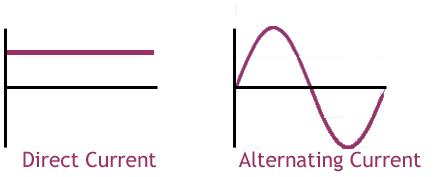• The current in DC circuits is moving in a constant direction. The amount of current can change, but it will always flow from one point to another. In alternating current, the charges move in one direction for a very short time, and then they reverse direction. This happens over and over again.
Offer running on EduRev: Apply code STAYHOME200 to get INR 200 off on our premium plan EduRev Infinity!

## Famous Books for UPSC Exam (Summary & Tests)

51 videos|371 docs|146 tests

,

,

,

,

,

,

,

,

,

,

,

,

,

,

,

,

,

,

,

,

,

;